## Cipher## May 15, 2017

### Powers Boothe dies at 68 (Sin City, Agents of S.H.I.E.L.D.)

Quick decode on the death of Powers Boothe (or retirement), this one looks very suspicious, if he was killed it was planned for a while but at the moment I'm leaning towards retirement, he supposedly died of natural causes in his sleep at the age of 68Powers = 7+6+5+5+9+1 = 33 (Full Reduction) dies on Sunday = 8+6+4+5+8+2 = 33 (Reverse Reduction)
Boothe = 2+6+6+2+8+5 = 29 (Full Reduction) died on a date with 29 numerology (5+14+2+0+1+7)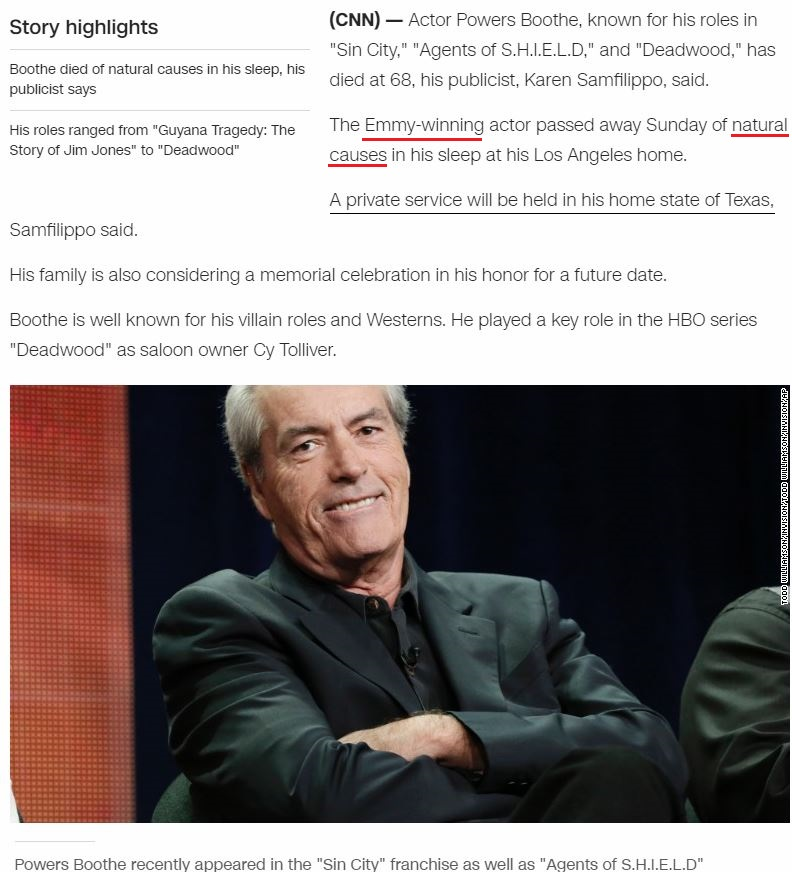There are very interesting connections to Sin City that is mentioned in this article, in the movie he plays the main antagonist called "Senator Roark" = 1+5+5+1+2+6+9 + 9+6+1+9+2 = 56 (Full Reduction) and he died at "Sixty eight" = 1+9+6+2+7 + 5+9+7+8+2 = 56 (Full Reduction) on a date with 56 numerology (5+14+20+17)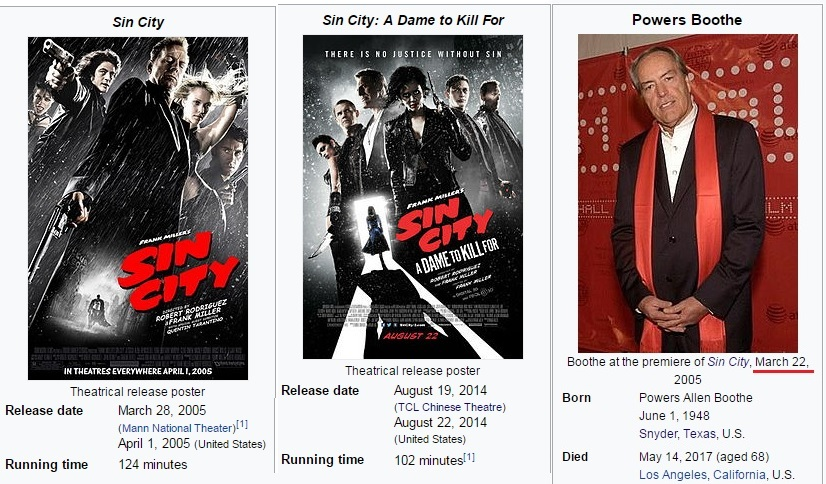Between Sin City movie releases we find 3430 days (7x7x7 = 343),  "Sin City" = 1+9+5 + 3+9+2+7 = 36 (Full Reduction) - Boothe died on a date with 36 numerology (5+14+17)From the release of Sin City: A Dame to Kill for on 8/22/2014 to Boothe's next birthday is 9 months and 11 daysMeasuring to his death from release of the sequel we find something even more interesting2 years 8 months 22 days from 8/22/2014, also some would say that 996 can be rearranged into 666, August 22nd is 234th day of the year (234+432 = 666), also they mention that Boothe was involved with "Agents of Shield" = 26+20+22+13+7+8 + 12+21 + 8+19+18+22+15+23 = 234 (Reverse Ordinal)

There's some 44 coding popping up and it goes well with "Agents of SHIELD" = 1+7+5+14+20+19 + 15+6 + 19+8+9+5+12+4 = 144 (English Ordinal)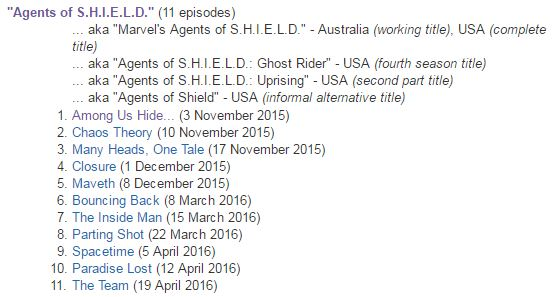He appeared on Agents of Shield for the last time on 4/19/2016, 44 days before his next birthday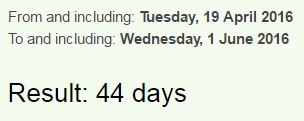Forty four = 6+15+18+20+25 + 6+15+21+18 = 144 (English Ordinal)
His birthday is also falling on a date with 44 numerology this year (6+1+20+17 = 44), Kill = 44 (English Ordinal)Execution 44 (Full Reduction)
Sin City: A Dame to Kill for came out on a date with 44 numerology as well (8+22+14)

CNN mentions that he's Emmy Winning actor and from his death to Emmy Awards this year we find 4 months and 4 days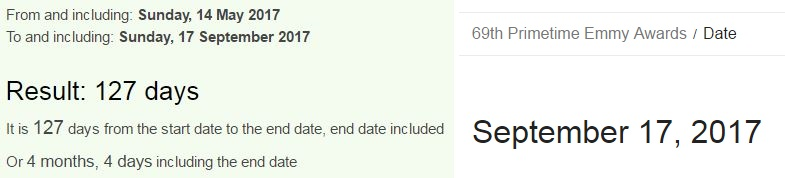Powers Boothe = 7+6+5+5+9+10 + 2+6+6+2+8+5 = 71 (Single Reduction)
44 and 71 are the only 2 numbers that sum to 144 when written out - Seventy one = 19+5+22+5+14+20+25 + 15+14+5 = 144 (English Ordinal), he was also starring in a TV show called "Deadwood" = 4+5+1+4+23+15+15+4 = 71 (English Ordinal)